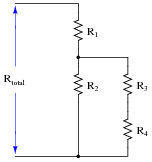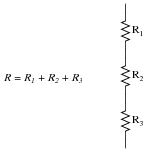# Algebraic Substitution for Electric Circuits

## Mathematics for Electronics

• #### Question 1

There are two basic Ohm’s Law equations: one relating voltage, current, and resistance; and the other relating voltage, current, and power (the latter equation is sometimes known as Joule’s Law rather than Ohm’s Law):

 E = I R

 P = I E

In electronics textbooks and reference books, you will find twelve different variations of these two equations, one solving for each variable in terms of a unique pair of two other variables. However, you need not memorize all twelve equations if you have the ability to algebraically manipulate the two simple equations shown above.

Demonstrate how algebra is used to derive the ten öther” forms of the two Ohm’s Law / Joule’s Law equations shown here.

• #### Question 2

The Q factor of a series inductive circuit is given by the following equation:

$$Q = \frac {X_L}{R_{series}}$$

Likewise, we know that inductive reactance may be found by the following equation:

$$X_L = 2\pi f L$$

We also know that the resonant frequency of a series LC circuit is given by this equation:

$$f_r = \frac {1}{2 \pi \sqrt{LC}}$$

Through algebraic substitution, write an equation that gives the Q factor of a series resonant LC circuit exclusively in terms of L, C, and R, without reference to reactance (X) or frequency (f).

• #### Question 3

We know that the current in a series circuit may be calculated with this formula:

 I = Etotal Rtotal

We also know that the voltage dropped across any single resistor in a series circuit may be calculated with this formula:

 ER = I R

Combine these two formulae into one, in such a way that the I variable is eliminated, leaving only ER expressed in terms of Etotal, Rtotal, and R.

• #### Question 4

Substitution is a technique whereby we let a variable represent (stand in the place of) another variable or an expression made of other variables. One application where we might use substitution is when we must manipulate an algebraic expression containing a lot of similar-looking variables, as is often the case with science problems.

Take this series-parallel resistor circuit for example:The equation expressing total resistance as a function of the four resistor values looks like this:

$$R_{total} = R_1 + \frac{R_2 (R_3 + R_4)}{R_2 + R_3 +R_4}$$

Now imagine being asked to manipulate this equation to solve for R3. When the only visual feature distinguishing each of the variables is the subscript (total, 1, 2, 3, or 4), it becomes very easy to lose track of where one is in the algebraic manipulation. A very common mistake is to exchange or needlessly repeat subscripts during the process, effectively mis-placing one or more variables. To help avoid such mistakes, you may substitute different letter variables for Rtotal, R1, R2, R3, and R4 like this:

 Substitution table

 Original variable New variable Rtotal y R1 a R2 b R3 c R4 d

$$y = a + \frac{b(c+d)}{b + c + d}$$

After doing the algebraic manipulation to solve for c (R3), the equation looks like this:

$$c = \frac{(y-a)(b+d)-bd}{a+b-y}$$

Back-substitute the original R variables in place of a, b, c, d, and y as you see them in the above equation to arrive at a form that directly relates to the schematic diagram.

• #### Question 5

Substitution is a technique whereby we let a variable represent (stand in the place of) another variable or an expression made of other variables. One application where we might use substitution is when we must manipulate an algebraic expression containing multiple instances of the same sub-expression. For example, suppose we needed to manipulate this equation to solve for c:

$$1 = \frac {a+b(d^2-f^2)+c}{d^2-f^2}$$

The sub-expression d2 − f2 appears twice in this equation. Wouldn’t it be nice if we had something simpler to put in its place during the time we were busy manipulating the equation, if for no other reason than to have less variables to write on our paper while showing all the steps to our work? Well, we can do this!

Substitute the variable x for the sub-expression d2 − f2, and then solve for c. When you are done manipulating the equation, back-substitute d2 − f2 in place of x.

• #### Question 6

Substitution is the term we give to the mathematical equivalence of one variable to one or more other variables in an expression. It is a fundamental principle used to combine two or more equations into a single equation (among other things).

For example, we know that the formula for calculating current in a simple one-resistor circuit is as follows:We also know that the total resistance (R) of a three-resistor series circuit is as follows:Combine these two equations together using substitution so that we have a single equation for calculating current I in a three-resistor series circuit given the source voltage V and each resistance value R1, R2, and R3:In other words, you need to have as your answer a single equation that begins with “I = ” and has all the variables V, R1, R2, and R3 on the other side of the ëqual” sign.

• #### Question 7

We know that the voltage in a parallel circuit may be calculated with this formula:

$$E = I_{total} \ R_{total}$$

We also know that the current through any single resistor in a parallel circuit may be calculated with this formula:

$$I_R = \frac {E}{R}$$

Combine these two formulae into one, in such a way that the E variable is eliminated, leaving only IR expressed in terms of Itotal, Rtotal, and R.

• #### Question 8

Suppose we only knew the emitter and base currents for an operating transistor and wished to calculate β from that information. We would need a definition of beta cast in terms of IE and IB instead of IC and IB.

Apply algebraic substitution to the formula $$\beta = \frac {I_C}{I_B}$$ so that beta (β) is defined in terms of IE and IB. You may find the following equation helpful in your work:

$$I_E = I_C + I_B$$

• #### Question 9

The resistance of a piece of copper wire at temperature T (in degrees Celsius) is given by the following formula:

$$R_T = R_o \ [1 + 0.004041(T − 20)]$$

Suppose you wished to alter this formula so it could accept values for T in units of degrees Fahrenheit instead of degrees Celsius. Suppose also that the only formula you are able to find for converting between Fahrenheit (TF) and Celsius (TC) is this one:

$$T_F = T_C (\frac{9}{5})+ 32$$

Combined these two formulae into one solving for the resistance of a copper wire sample (RT) at a specific temperature in degrees Celsius (TC), given the specimen’s “reference” resistance (Ro) at 20o Celsius (room temperature).

• #### Question 10

A bipolar junction transistor parameter similar to β is älpha,” symbolized by the Greek letter α. It is defined as the ratio between collector current and emitter current:

$$a = \frac{I_C}{I_E}$$

Apply algebraic substitution to this formula so that alpha is defined as a function of beta: α = f(β). In other words, substitute and manipulate this equation until you have alpha by itself on one side and no variable except beta on the other.

$$\beta = \frac{I_C}{I_B} \ \ \ \ \ \ \ \ \ \ \ \ \ \ \ I_E=I_C+I_B$$

• #### Question 11

The Q, or quality factor, of an inductor circuit is defined by the following equation, where Xs is the series inductive reactance and Rs is the series resistance:

$$Q = \frac{X_s}{R_s}$$

We also know that we may convert between series and parallel equivalent AC networks with the following conversion equations:

$$R_s R_p = Z^2 \ \ \ \ \ \ \ \ \ \ \ \ \ \ \ \ X_sX_p = Z^2$$Series and parallel LR networks, if truly equivalent, should share the same Q factor as well as sharing the same impedance. Develop an equation that solves for the Q factor of a parallel LR circuit.

• #### Question 12

The equation relating probability of continued performance for a component or a system versus time may be expressed as follows:

 x = e−t / m

Where,

x = Probability (a number between 0 and 1, inclusive)

e = Euler’s constant ( ≈ 2.7182818)

t = Time of continuous operation

m = Mean Time Between Failure of the component or system

The unit of time for both t and m must be the same. That is, if t is measured in years, then m must also be expressed in years or else the equation will give very misleading answers.

Suppose, though, we were given m in years, and the operating time t in days. Substitute the relationship td = 365 ty into the reliability equation so that we will have a new equation that can take t in days (td) and m in years, and still provide the correct answer.

• #### Question 13

The voltage-dependent capacitance of a varactor diode is given by the following equation:

$$C_j = \frac {C_o}{\sqrt{2V+1}}$$

Where,

CJ = Junction capacitance

Co = Junction capacitance with no applied voltage

V = Applied reverse junction voltage

Combine this equation with the standard equation for frequency in a resonant LC circuit to arrive at a new equation giving resonant frequency in terms of Co, V, and L.